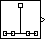Documentation

Discrete Impulse

Generate discrete impulse

• Library:
• DSP System Toolbox / SourcesDescription

The Discrete Impulse block generates an impulse (the value 1) at output sample D+1, where you specify D using the Delay parameter (D0). All output samples preceding and following sample D+1 are zero.

When D is a length-N vector, the block generates an M-by-N matrix output representing N distinct channels, where you specify frame size M using the Samples per frame parameter. The impulse for the ith channel appears at sample D(i)+1.

The Sample time parameter value, Ts, specifies the output signal sample period. The resulting frame period is MTs.

Ports

Output

expand all

Output signal containing a discrete impulse at output sample D(i)+1, where D is a scalar or vector specified by the Delay parameter. For more information, see Description.

Data Types: single | double | int8 | int16 | int32 | uint8 | uint16 | uint32 | Boolean | fixed point

Parameters

expand all

Main

The number of zero-valued output samples, D, preceding the impulse, specified as a scalar or vector of integer values, greater than or equal to zero. A length-N vector specifies an N-channel output.

The sample period, Ts, of the output signal specified as a positive finite scalar. The output frame period is MTs.

The number of samples, M, in each output frame, specified as a positive integer scalar.

Data Types

Specify the output data type for this block. You can select one of the following:

• A rule that inherits a data type, for example, Inherit: Inherit via back propagation. When you select this option, the output data type and scaling matches that of the next downstream block.

• A built-in data type, such as double

• An expression that evaluates to a valid data type, for example, fixdt(1,16)

For help setting data type parameters, display the Data Type Assistant by clicking the button.

Block Characteristics

 Data Types Boolean | double | fixed point | integer | single Direct Feedthrough no Multidimensional Signals no Variable-Size Signals no Zero-Crossing Detection no